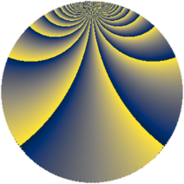# Properties

 Label 900.3.bbLevel $900$ Weight $3$ Character orbit 900.bb Rep. character $\chi_{900}(19,\cdot)$ Character field $\Q(\zeta_{10})$ Dimension $592$ Sturm bound $540$

# Related objects

## Defining parameters

 Level: $$N$$ $$=$$ $$900 = 2^{2} \cdot 3^{2} \cdot 5^{2}$$ Weight: $$k$$ $$=$$ $$3$$ Character orbit: $$[\chi]$$ $$=$$ 900.bb (of order $$10$$ and degree $$4$$) Character conductor: $$\operatorname{cond}(\chi)$$ $$=$$ $$100$$ Character field: $$\Q(\zeta_{10})$$ Sturm bound: $$540$$

## Dimensions

The following table gives the dimensions of various subspaces of $$M_{3}(900, [\chi])$$.

Total New Old
Modular forms 1472 608 864
Cusp forms 1408 592 816
Eisenstein series 64 16 48

## Trace form

 $$592q + 5q^{2} - 3q^{4} + 10q^{5} + 20q^{8} + O(q^{10})$$ $$592q + 5q^{2} - 3q^{4} + 10q^{5} + 20q^{8} - 13q^{10} - 10q^{13} - 15q^{14} - 63q^{16} + 10q^{17} - 7q^{20} + 70q^{22} - 30q^{25} + 66q^{26} - 85q^{28} - 14q^{29} + 69q^{34} - 20q^{37} + 155q^{38} - 210q^{40} - 94q^{41} - 70q^{44} - 95q^{46} + 3712q^{49} + 463q^{50} + 480q^{52} + 80q^{53} + 60q^{56} + 370q^{58} + 14q^{61} + 330q^{62} + 12q^{64} + 140q^{65} - 418q^{70} - 10q^{73} - 304q^{74} + 80q^{76} + 500q^{77} - 82q^{80} - 40q^{85} + 165q^{86} - 170q^{88} - 384q^{89} + 940q^{92} + 325q^{94} + 190q^{97} + 340q^{98} + O(q^{100})$$

## Decomposition of $$S_{3}^{\mathrm{new}}(900, [\chi])$$ into newform subspaces

The newforms in this space have not yet been added to the LMFDB.

## Decomposition of $$S_{3}^{\mathrm{old}}(900, [\chi])$$ into lower level spaces

$$S_{3}^{\mathrm{old}}(900, [\chi]) \cong$$ $$S_{3}^{\mathrm{new}}(100, [\chi])$$$$^{\oplus 3}$$$$\oplus$$$$S_{3}^{\mathrm{new}}(300, [\chi])$$$$^{\oplus 2}$$